# Hölder condition

(diff) ← Older revision | Latest revision (diff) | Newer revision → (diff)

An inequality in which the increment of a function is expressed in terms of the increment of its argument. A function, defined in a domainof an-dimensional Euclidean space, satisfies the Hölder condition at a point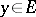with index(of order), where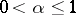, and with coefficient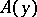, if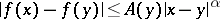(1)

for allsufficiently close to. One says thatsatisfies the (isotropic) Hölder condition with indexon a set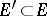if (1) is satisfied for all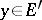. If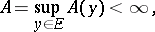the Hölder condition is called uniform on, whileis called the Hölder coefficient ofon. Functions satisfying a Hölder condition are often referred to as Hölder continuous. The quantityis called the Hölder-semi-norm of a bounded functionon the set. The Hölder semi-norm as a function ofis logarithmically convex: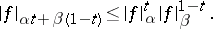The non-isotropic Hölder condition is introduced similarly to the condition (1), and has the form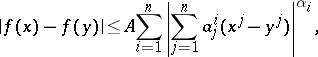where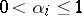and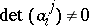. Functions which satisfy the non-isotropic Hölder condition are continuous and have Hölder index,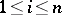, in the direction of the covector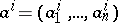.

A condition of the form (1) was introduced by R. Lipschitz in 1864 for functions of one real variable in the context of a study of trigonometric series. In such a case the Hölder condition is often called the Lipschitz condition of orderwith Lipschitz constant. For functions of,, real variables the Hölder condition was introduced by O. Hölder in his studies of the differentiability properties of the Newton potential.

The Hölder condition can be naturally extended to the case of mappings of metric spaces. One says that a mappingof a metric spaceinto a metric spacesatisfies the Hölder condition with indexand coefficient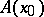at a pointif there exists a neighbourhoodofsuch that for any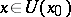the inequality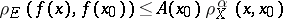is valid. Here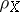andare the metrics of the spacesand. The Hölder condition on a set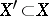, the uniform Hölder condition onand the Hölder-semi-norm are introduced in a similar manner.

A vector space of functions which satisfy any Hölder condition is a Hölder space.

How to Cite This Entry:
Hölder condition. Encyclopedia of Mathematics. URL: http://encyclopediaofmath.org/index.php?title=H%C3%B6lder_condition&oldid=16871
This article was adapted from an original article by L.P. Kuptsov (originator), which appeared in Encyclopedia of Mathematics - ISBN 1402006098. See original article